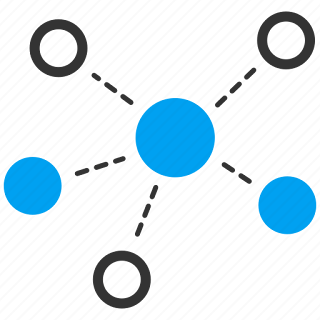# Types of Graph Data Structure# Types of Graph Data Structure

Graphs are a fundamental data structure used in computer science to model relationships between objects. A graph consists of a set of vertices (also known as nodes) and a set of edges, which connect the vertices. There are several different types of graphs, each with its own unique characteristics.

## Undirected Graph

An undirected graph is a graph in which the edges have no direction. This means that the edges connect vertices in both directions. In other words, if there is an edge between vertex A and vertex B, then there is also an edge between vertex B and vertex A. An example of an undirected graph is shown below:## Directed Graph

A directed graph is a graph in which the edges have a direction. This means that the edges connect vertices in one direction only. In other words, if there is an edge from vertex A to vertex B, then there is no edge from vertex B to vertex A unless specifically defined. An example of a directed graph is shown below:## Weighted Graph

A weighted graph is a graph in which each edge has a weight or cost associated with it. This weight can represent the distance between two vertices, the time it takes to traverse the edge, or any other metric that is relevant to the problem being solved. An example of a weighted graph is shown below:## Connected Graph

A connected graph is a graph in which there is a path from any vertex to any other vertex. In other words, all vertices in the graph are reachable from any other vertex. An example of a connected graph is shown below:## Spanning Tree

A spanning tree is a subgraph of a graph that contains all the vertices of the graph and is a tree. A tree is a connected graph with no cycles. A spanning tree of a graph is a way of connecting all the vertices of the graph without creating any cycles. An example of a spanning tree for the previous connected graph is shown below:## Minimum Spanning Tree

A minimum spanning tree is a spanning tree of a weighted graph that has the smallest possible total weight. In other words, it is a way of connecting all the vertices of a weighted graph with the smallest possible cost. There are several algorithms that can be used to find the minimum spanning tree of a graph, including Prim's algorithm and Kruskal's algorithm. An example of a minimum spanning tree for the previous weighted graph is shown below:In conclusion, these are the different types of graph data structures along with their respective characteristics. Understanding the differences between these types of graphs is important when designing algorithms that make use of graphs as a data structure.